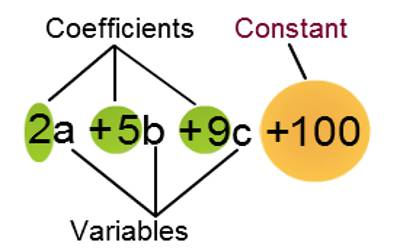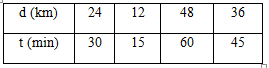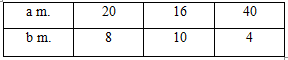Variable in Mathematics

In Mathematics, we usually deal with two types of quantities-Variable quantities ( or variables ) and Constant quantities ( or constants). If the value of a quantity remains unaltered under different situations, it is called a constant. On the contrary, if the value of a quantity changes under different situations, it is called a variable.

For example 4, 2.718, 22/7 etc. are constants while the speed of a train, the demand for a commodity, population of a town etc. are variables.

In problems relating to two or more variables, it is seen that the value of variable changes with the change in the value (or values) of the related variable (or variables). Suppose a train running at a uniform speed of v km./h. travels a distance of d km. in t hours. Obviously, if t remains unchanged then v increases or decreases according to as d increases or decreases. But if d remains unchanged, then v decreases or increases according to as ‘t’ increases or decreases. This shows that the change in the value of a variable may be accompanied differently with the change in the values of related variables. Such relationship with regards to the change in the value of a variable when the values of the related variables change is termed as a variation.We will discuss such variations, which are classified into three types:

(1) Direct Variation

(2) Inverse Variation and

(3) Joint Variation.

Direct Variation:

If two variables A and B are so related that when A increases ( or decreases ) in a given ratio, B also increases ( or, decreases ) in the same ratio, then A is said to vary directly as B ( or, A is said to vary as B ). This is symbolically written as, A ∝ B (read as ‘A varies as B’ ). Suppose a train moving at a uniform speed travels d km. in t minutes. Now, consider the following table:Inverse Variation:

A variable quantity A is said to vary inversely as another variable quantity B when A varies as the reciprocal of B i.e. when A varies as 1/B

Thus, if A varies inversely as B, we write A ∝ 1/B or, A = m ∙ (1/B) or, AB = m where m (≠ 0) is the constant of variation. Hence, if one variable varies inversely as another, then the product of the corresponding values of the variables is constant.

Conversely, if AB = k where A and B are variables and k is a constant, then A ∝ 1/B Hence, if the product of the corresponding values of two variables is constant, then one quantity varies inversely as another.

Again, if A varies inversely as & then AB = constant; but AB = constant implies that when A increases in a given ratio, B decreases in the same ratio and vice — versa. Thus, if two variables are so related that an increase (or decrease ) in the value of one variable in a certain ratio corresponds to a decrease (or increase) in the same ratio in the value of the other variable then one variable varies inversely as another.

Let a m. and b m. be the length and breadth r area 160 sq. m. Now, consider the following table:Joint Variation:

One variable quantity is said to vary jointly as a number of other variable quantities when it varies directly as their product. If the variable A varies directly as the product of the variables B, C and D, i.e., if.A ∝ BCD or A = kBCD (k = constant ), then A varies jointly as B, C, and D.

We know, the area of a triangle = ½ × base × altitude. Since ½ is a constant, hence area of a triangle varies oint1y as its base and altitude.

A is said to vary directly as B and inversely as C if A ∝ B ∙ 1/C or A = m ∙ B ∙ 1/C (m = constant of variation) i.e., if A varies jointly as B and 1/C.

If x men take y days to plough z acres of land, then x varies directly as z and inversely as y.

Information Source: